Скачать презентацию Section 2 2 Quadratic Functions Graphs of

535f73ae3d38e6d24227027051f184c0.ppt

• Количество слайдов: 30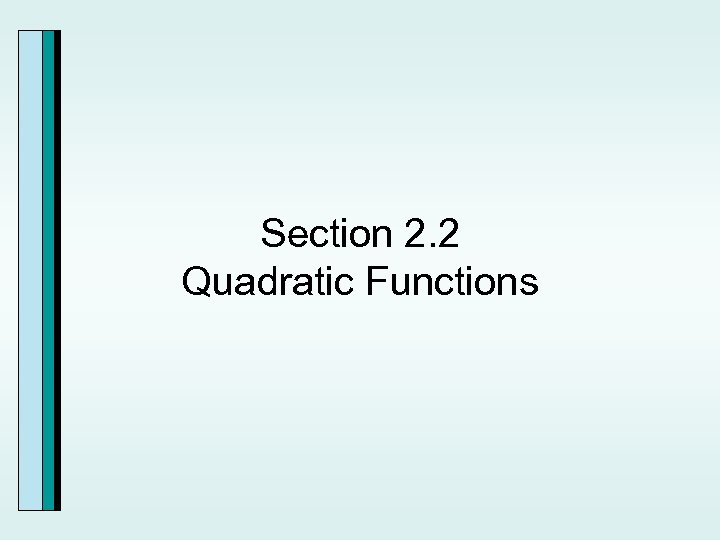Section 2. 2 Quadratic Functions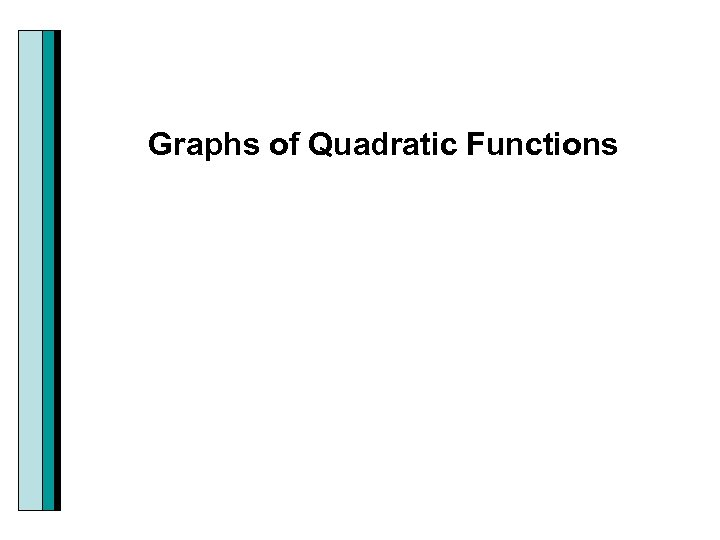Graphs of Quadratic Functions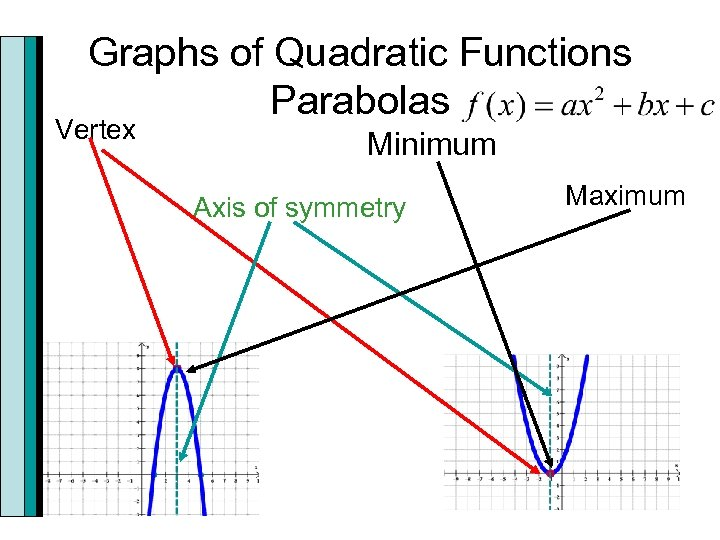Graphs of Quadratic Functions Parabolas Vertex Minimum Axis of symmetry Maximum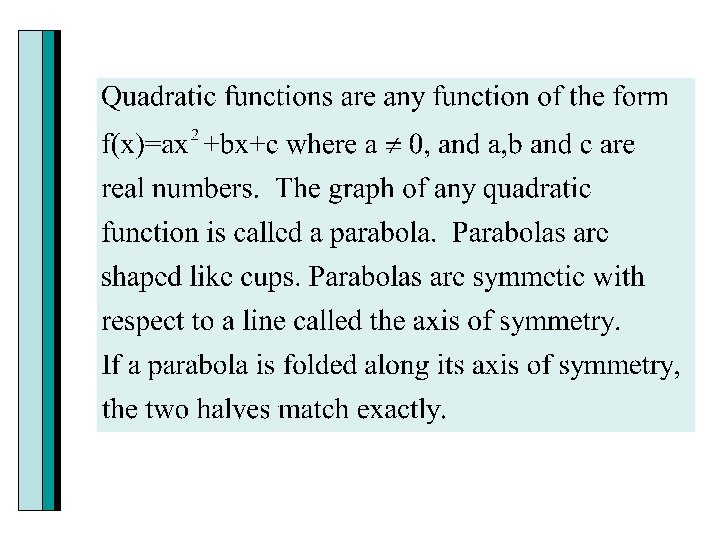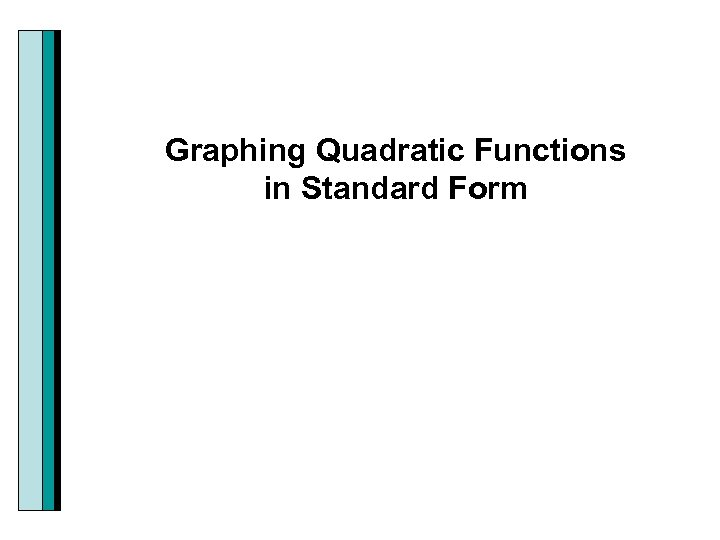Graphing Quadratic Functions in Standard Form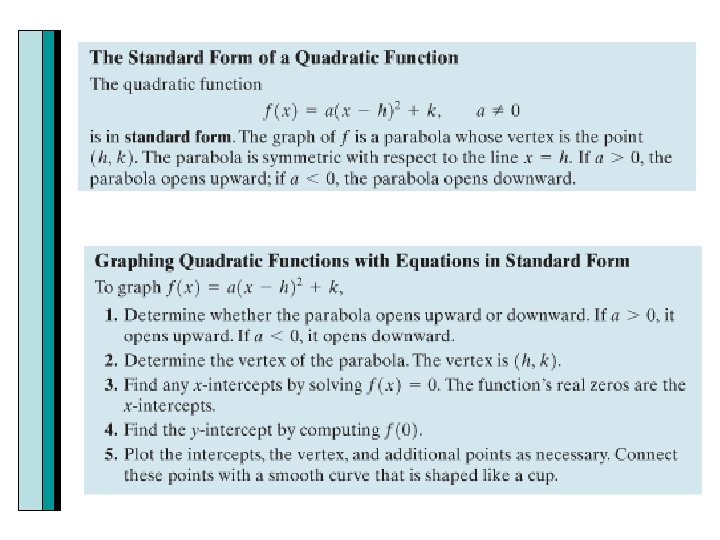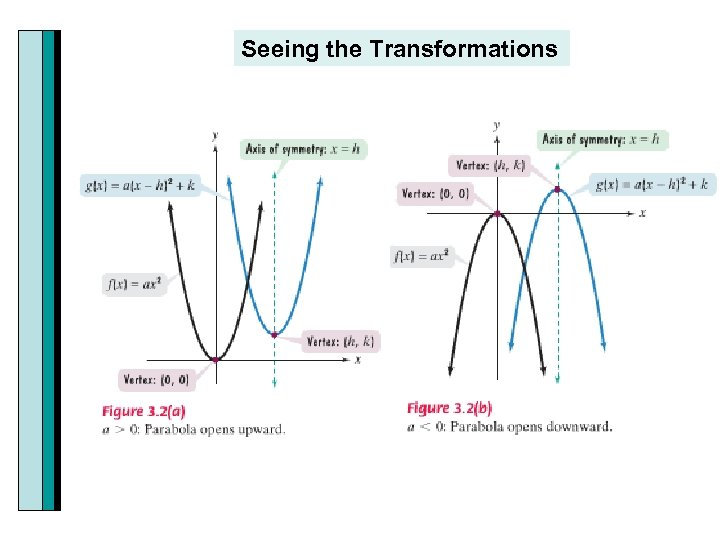Seeing the Transformations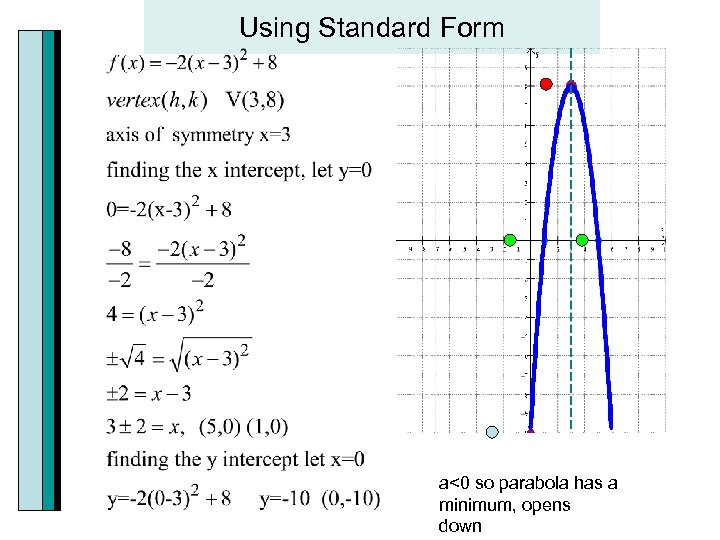Using Standard Form a<0 so parabola has a minimum, opens down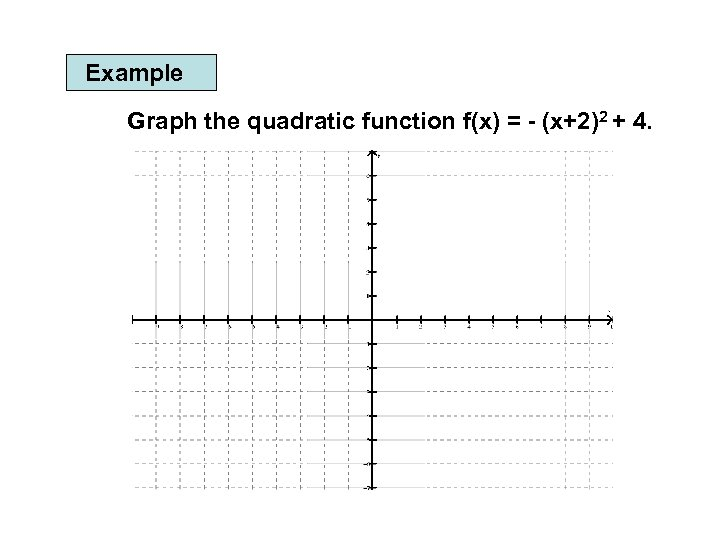Example Graph the quadratic function f(x) = - (x+2)2 + 4.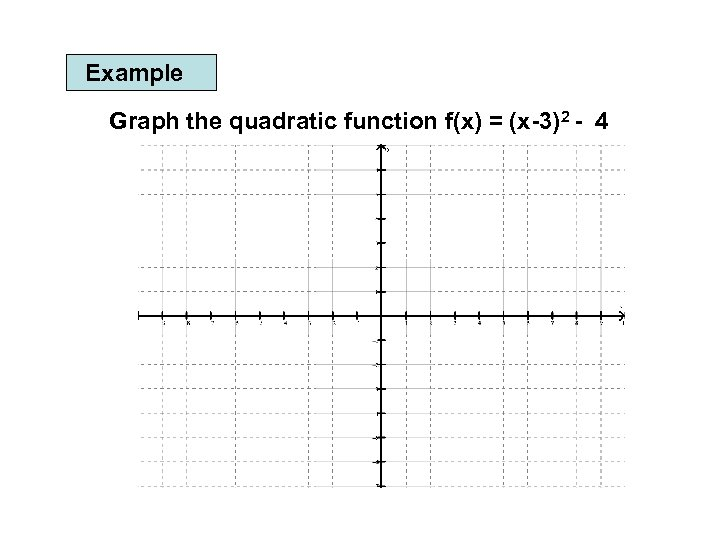Example Graph the quadratic function f(x) = (x-3)2 - 4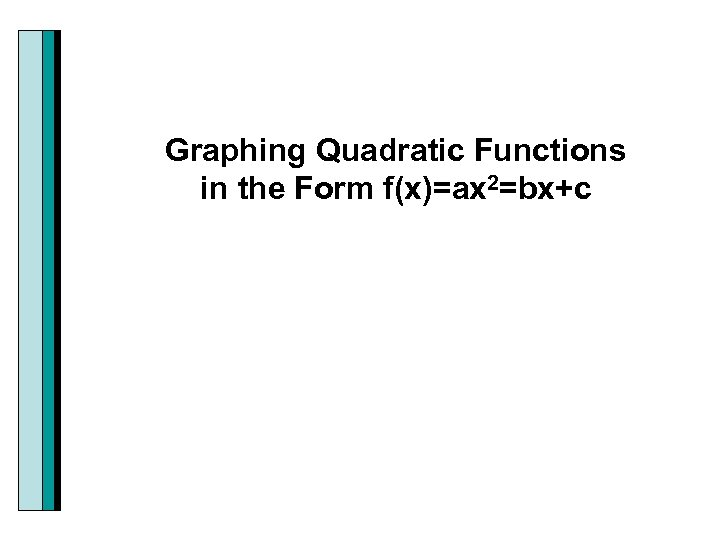Graphing Quadratic Functions in the Form f(x)=ax 2=bx+c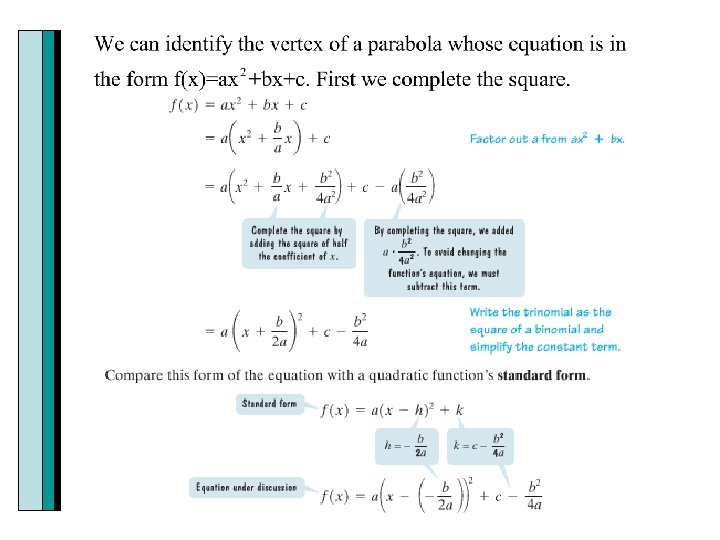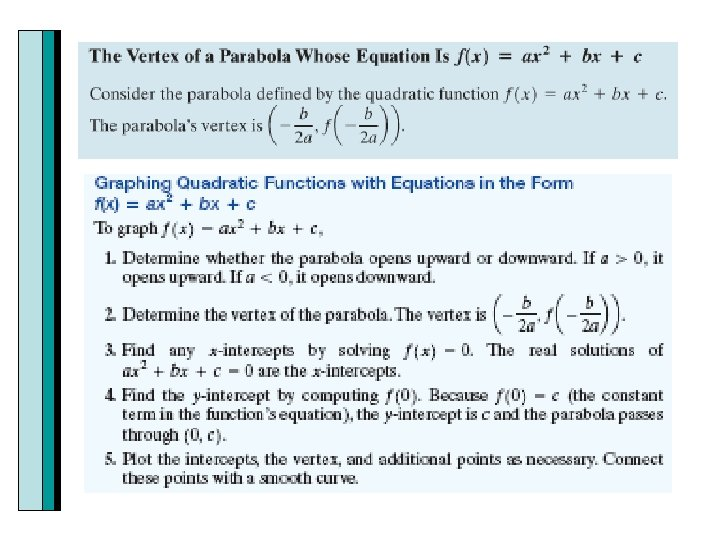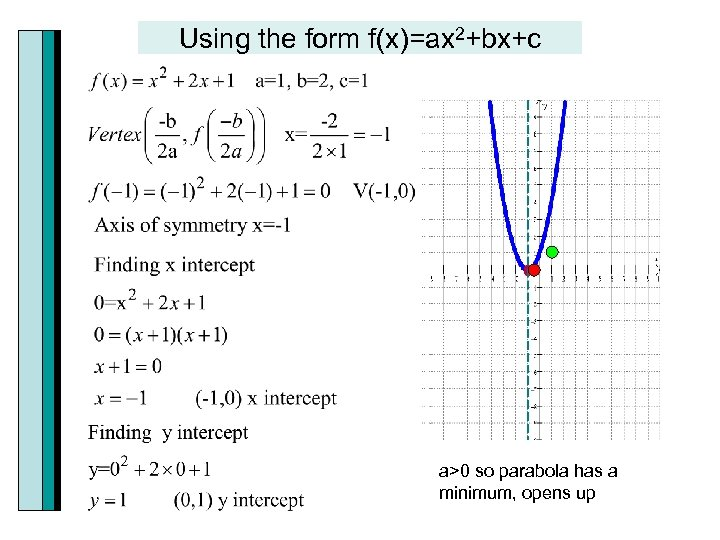Using the form f(x)=ax 2+bx+c a>0 so parabola has a minimum, opens up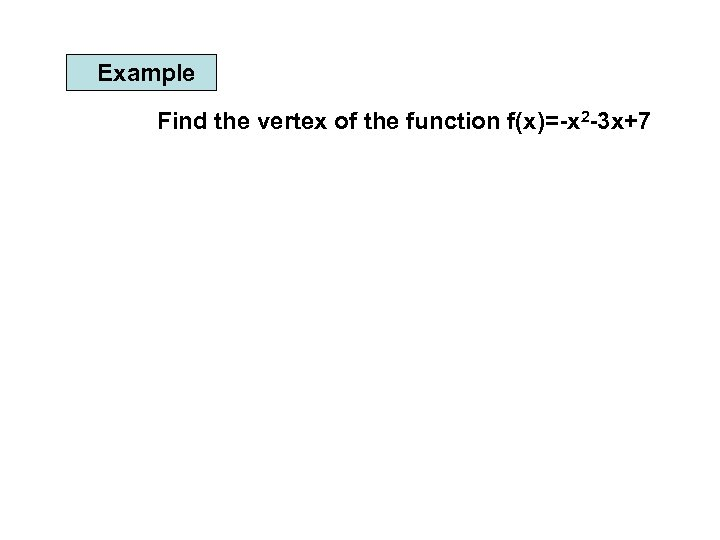Example Find the vertex of the function f(x)=-x 2 -3 x+7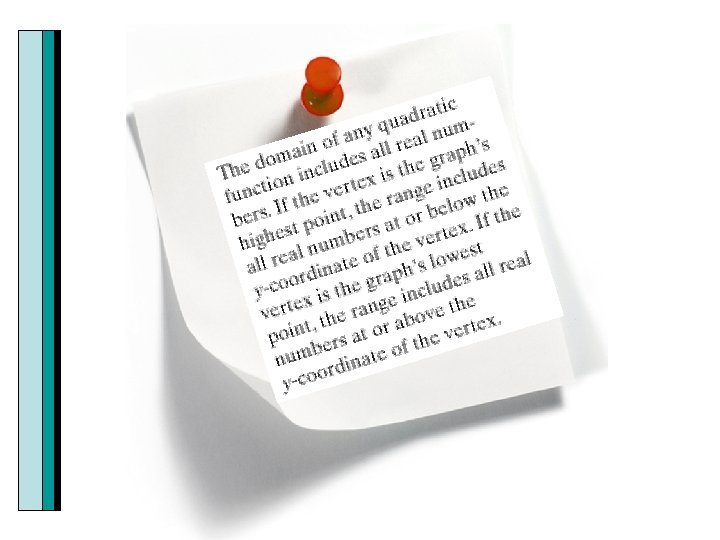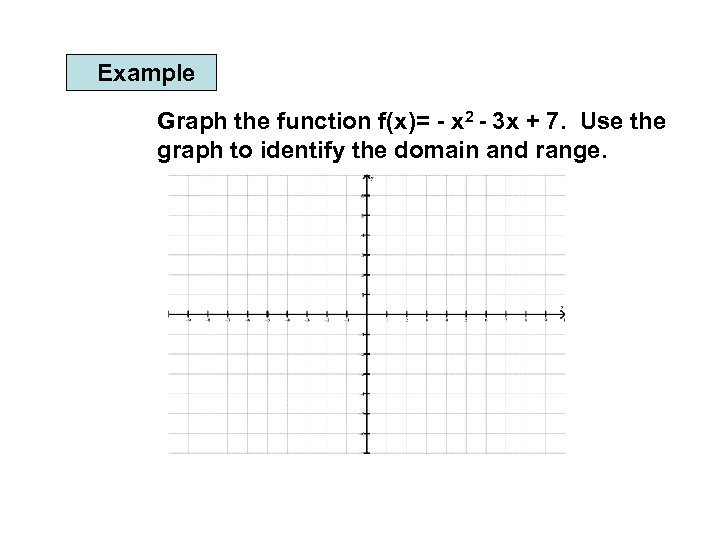Example Graph the function f(x)= - x 2 - 3 x + 7. Use the graph to identify the domain and range.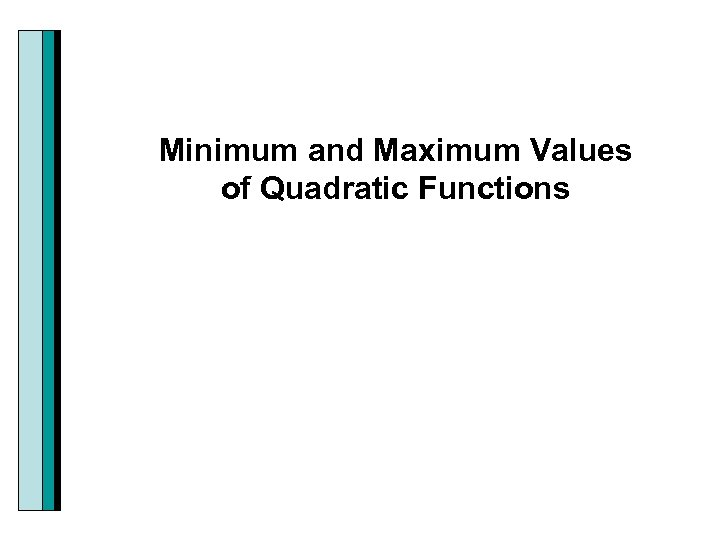Minimum and Maximum Values of Quadratic Functions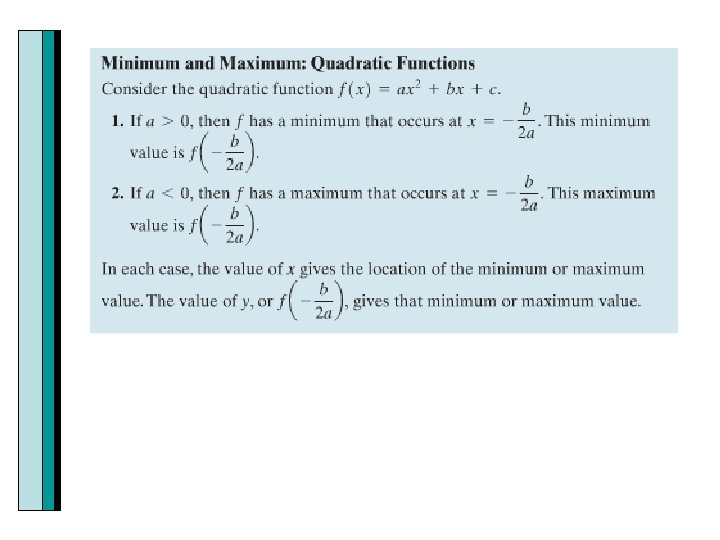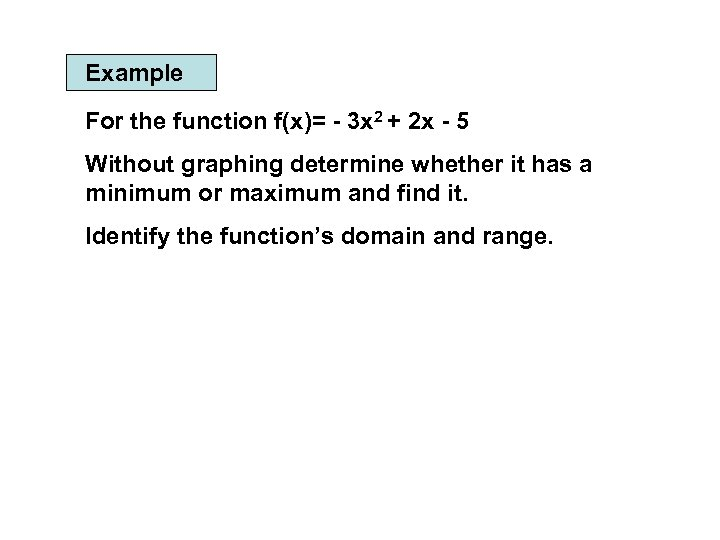Example For the function f(x)= - 3 x 2 + 2 x - 5 Without graphing determine whether it has a minimum or maximum and find it. Identify the function’s domain and range.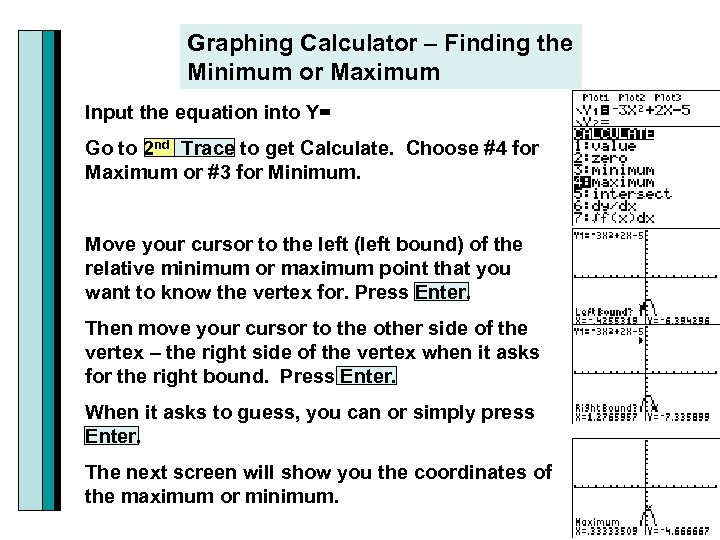Graphing Calculator – Finding the Minimum or Maximum Input the equation into Y= Go to 2 nd Trace to get Calculate. Choose #4 for Maximum or #3 for Minimum. Move your cursor to the left (left bound) of the relative minimum or maximum point that you want to know the vertex for. Press Enter. Then move your cursor to the other side of the vertex – the right side of the vertex when it asks for the right bound. Press Enter. When it asks to guess, you can or simply press Enter. The next screen will show you the coordinates of the maximum or minimum.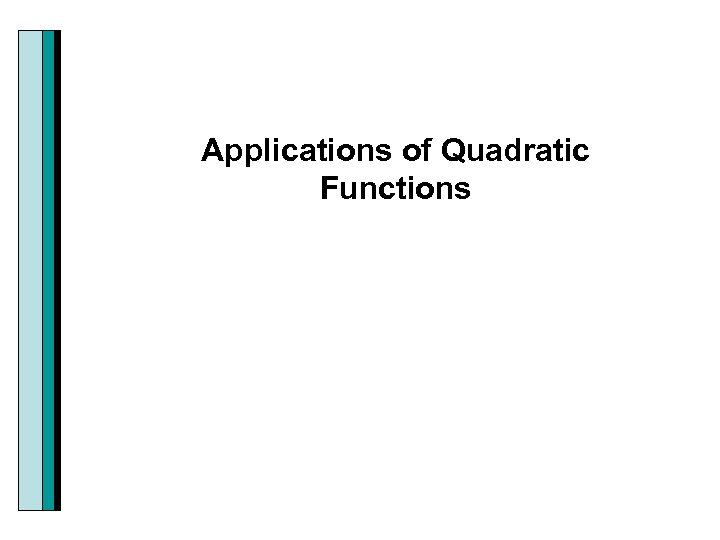Applications of Quadratic Functions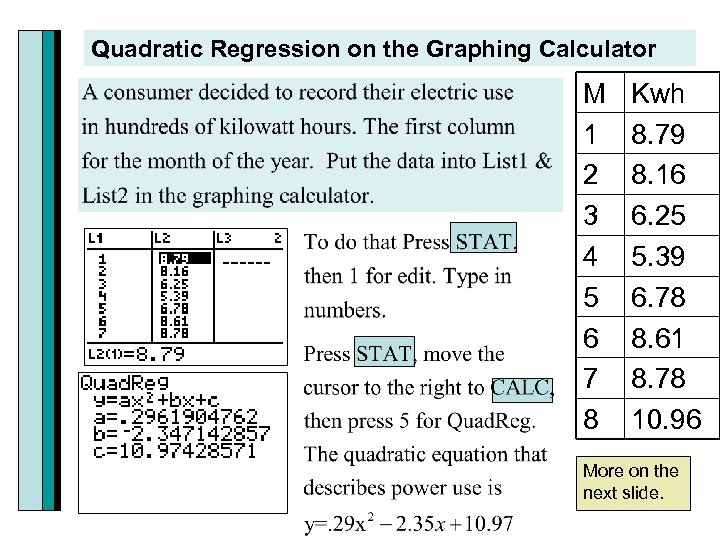Quadratic Regression on the Graphing Calculator M 1 2 3 4 5 6 7 8 Kwh 8. 79 8. 16 6. 25 5. 39 6. 78 8. 61 8. 78 10. 96 More on the next slide.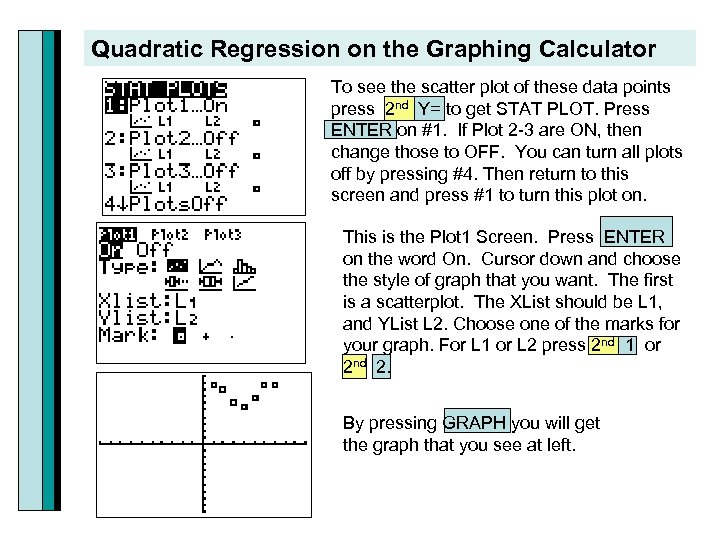Quadratic Regression on the Graphing Calculator To see the scatter plot of these data points press 2 nd Y= to get STAT PLOT. Press ENTER on #1. If Plot 2 -3 are ON, then change those to OFF. You can turn all plots off by pressing #4. Then return to this screen and press #1 to turn this plot on. This is the Plot 1 Screen. Press ENTER on the word On. Cursor down and choose the style of graph that you want. The first is a scatterplot. The XList should be L 1, and YList L 2. Choose one of the marks for your graph. For L 1 or L 2 press 2 nd 1 or 2 nd 2. By pressing GRAPH you will get the graph that you see at left.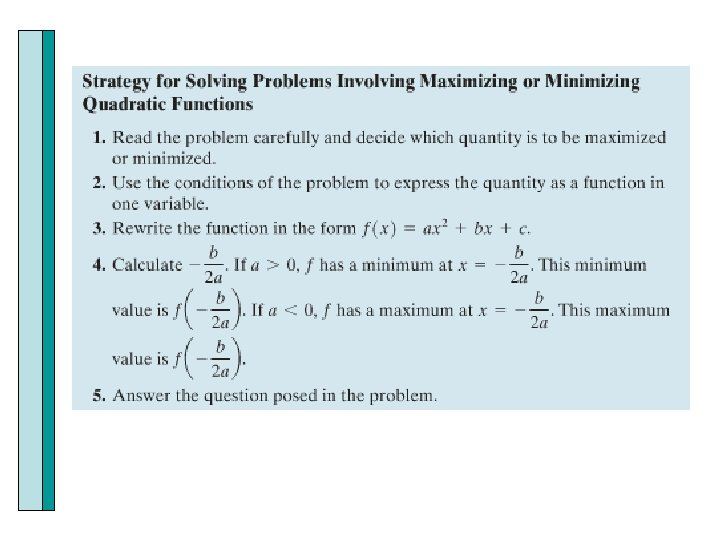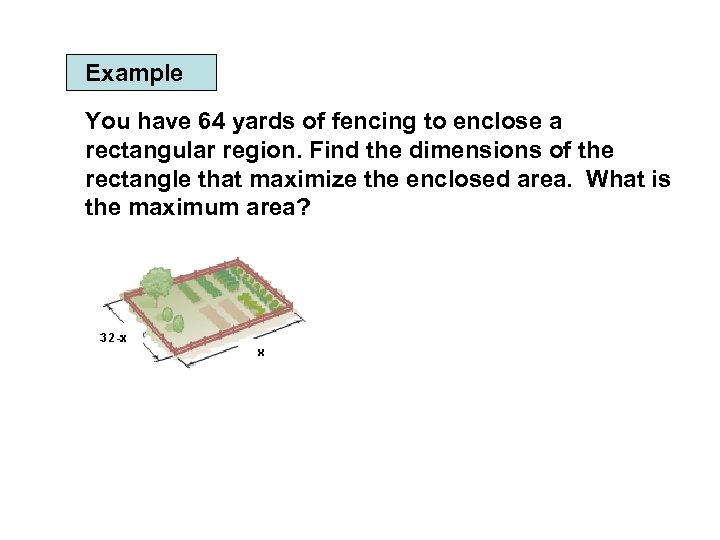Example You have 64 yards of fencing to enclose a rectangular region. Find the dimensions of the rectangle that maximize the enclosed area. What is the maximum area?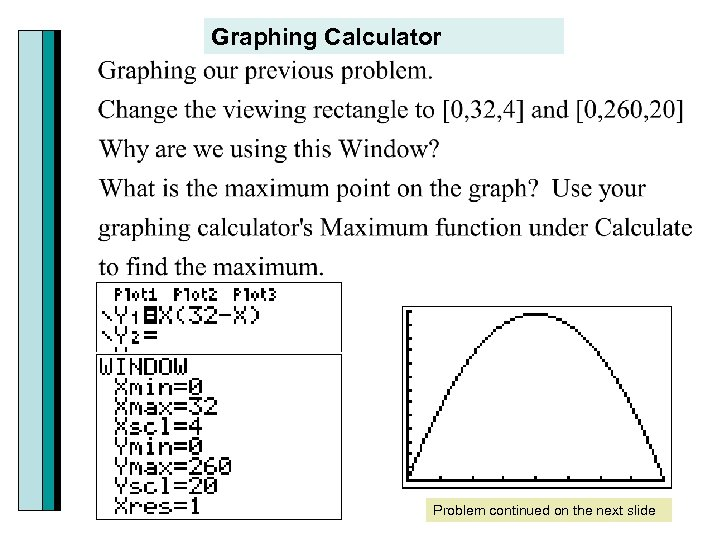Graphing Calculator Problem continued on the next slide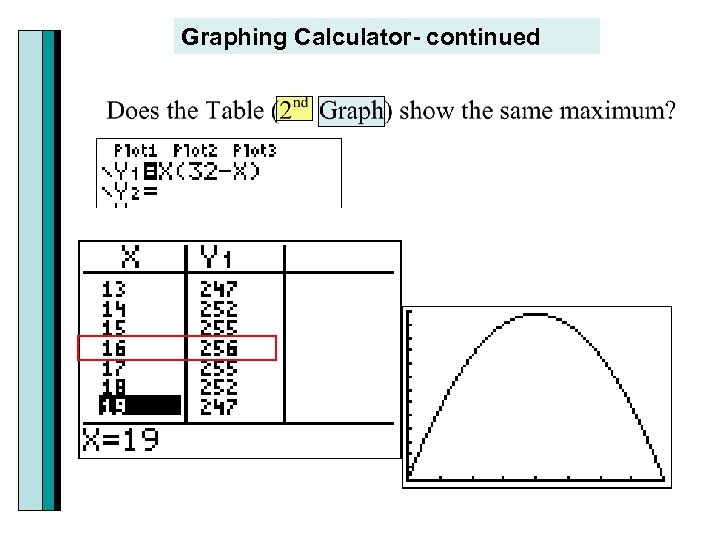Graphing Calculator- continued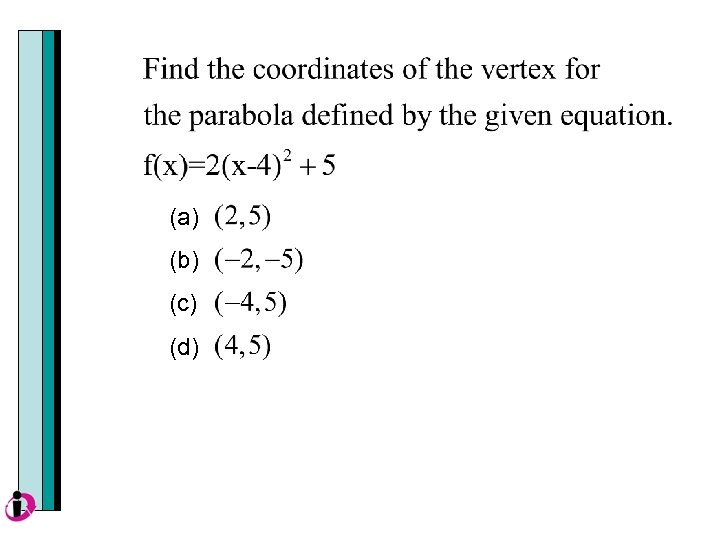(a) (b) (c) (d)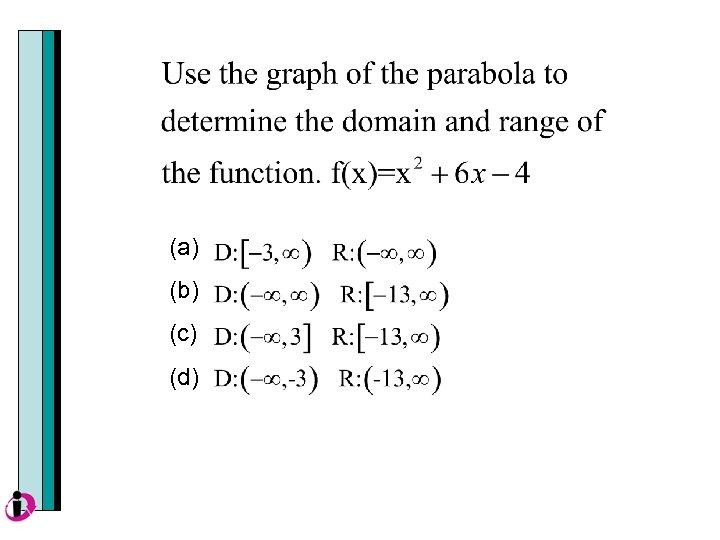(a) (b) (c) (d)